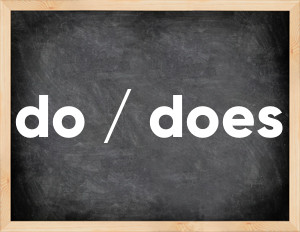# Do / does past tenseEnglish verb do / does [duː].
Relate to: irregular verbs.
3 forms of verb do / does: Infinitive (do / does), Past Simple - (did), Past Participle - (done).

## Forms of verb do / does in past tenses

👉 Forms of verb do / does in future and past simple and past participle.
❓ What is the past tense of do / does.

## Three forms of verb do / does

Base Form Past Simple Past Participle
do / does [duː]

did [dɪd]

done [dʌn]

## What is 2nd and 3rd form of do / does?

🎓 Base Form (Infinitive) do / does in Past Simple, Future Simple, Present Perfect, Past Perfect, Future Perfect?

• First form (V1) - do / does. (Present simple, Future Simple)
• Second form (V2) - did. (Past simple)
• Third form (V3) - done. (Present perfect, Past perfect)

## What are the past tense and past participle of do / does?

The past tense of do / does is: do / does in past simple is did. and past participle is done.

### What is the past tense of do / does?

The past tense of do / does is did.
The past participle of do / does is done.

### Verb Tenses

Past simple — do / does in past simple did. (V2)
Future simple — do / does in future simple do / does. (will + V1)
Present Perfect — do / does in present perfect tense done. (have\has + V3)
Past Perfect — do / does in past perfect tense done. (had + V3)

### do / does regular or irregular verb?

👉 Is do / does an irregular verb?? do / does is irregular verb.

## Examples of Verb do / does in Sentences

•   We do this job every 2 weeks. (Present Simple)
•   She does her singing lessons all the time. (Present Simple)
•   All they did was to lose 200 more soldiers. (Past Simple)
•   We have done our job, now it's your turn. (Present Perfect)
•   Check to see if the porridge is done. (Present Simple)
•   He did a lot for us when we were children. (Past Simple)
•   I always help my mum to do the dishes. (Present Simple)
•   What are you doing here? (Present Simple)
•   I will do my work if you pay me. (Future Simple)
•   I can't do it by myself! (Present Simple)

Along with do / does, words are popular kneel and happen.

Verbs by letter: , , , , , , , , , , , , , , , , , , , , , , , , .